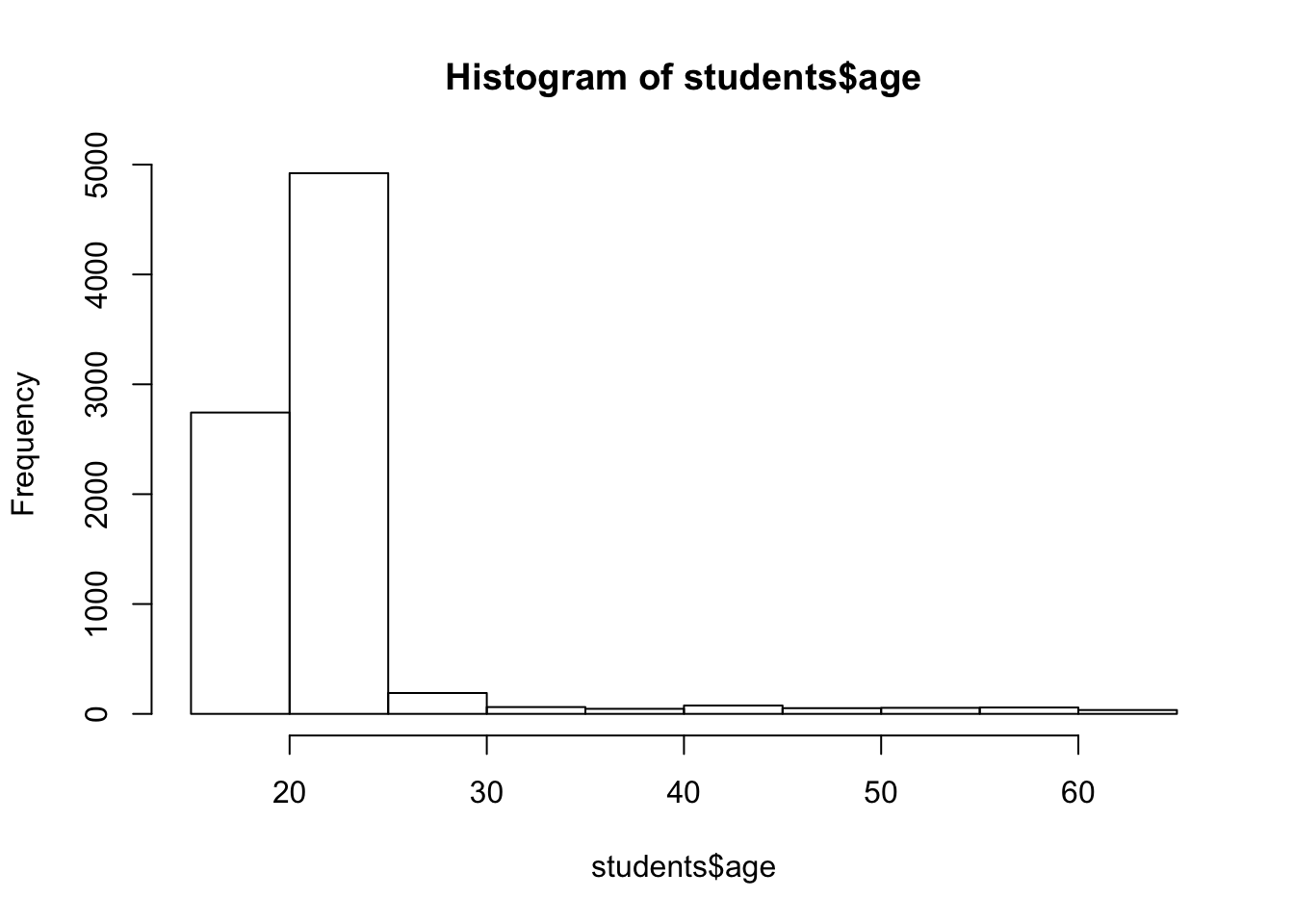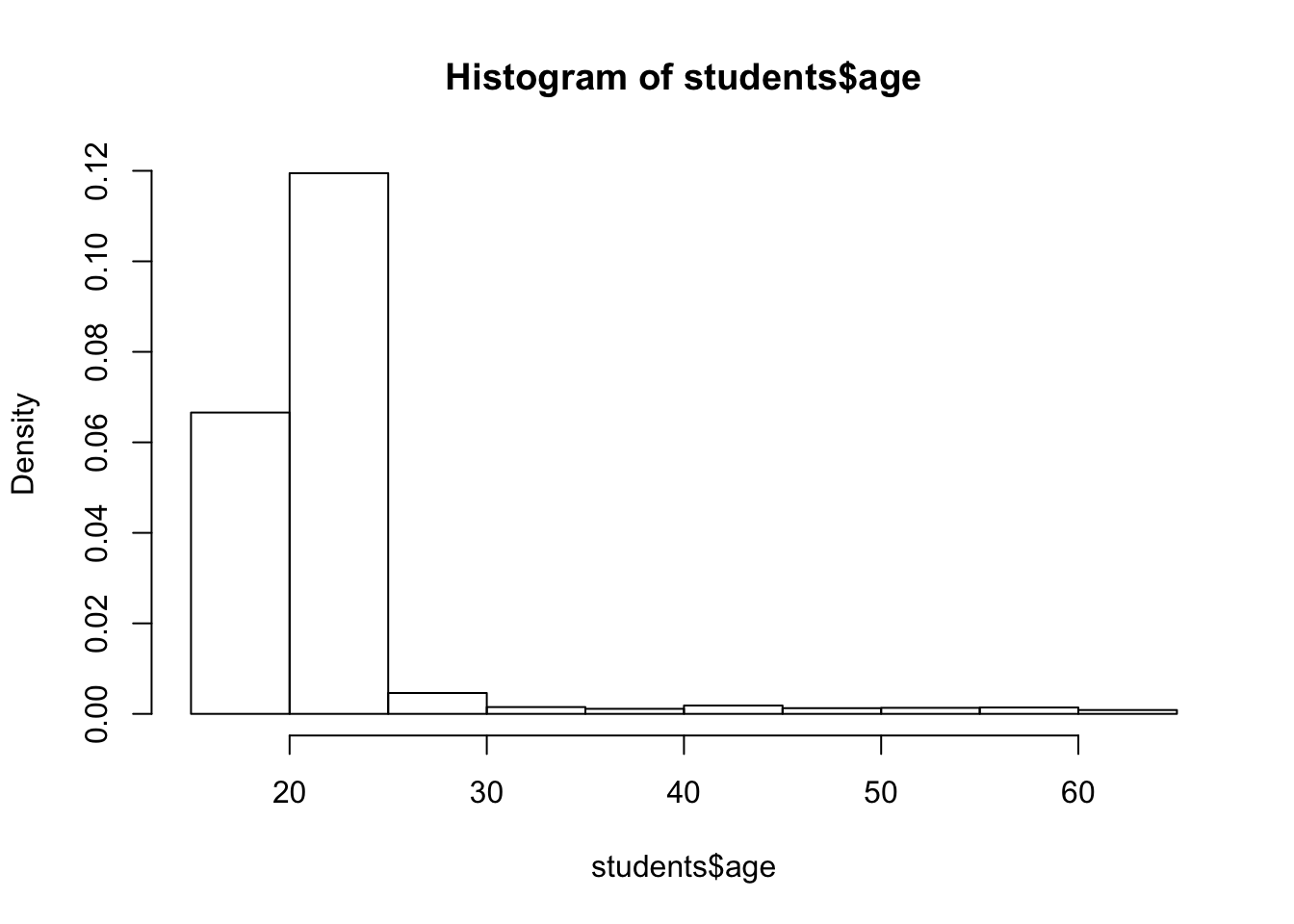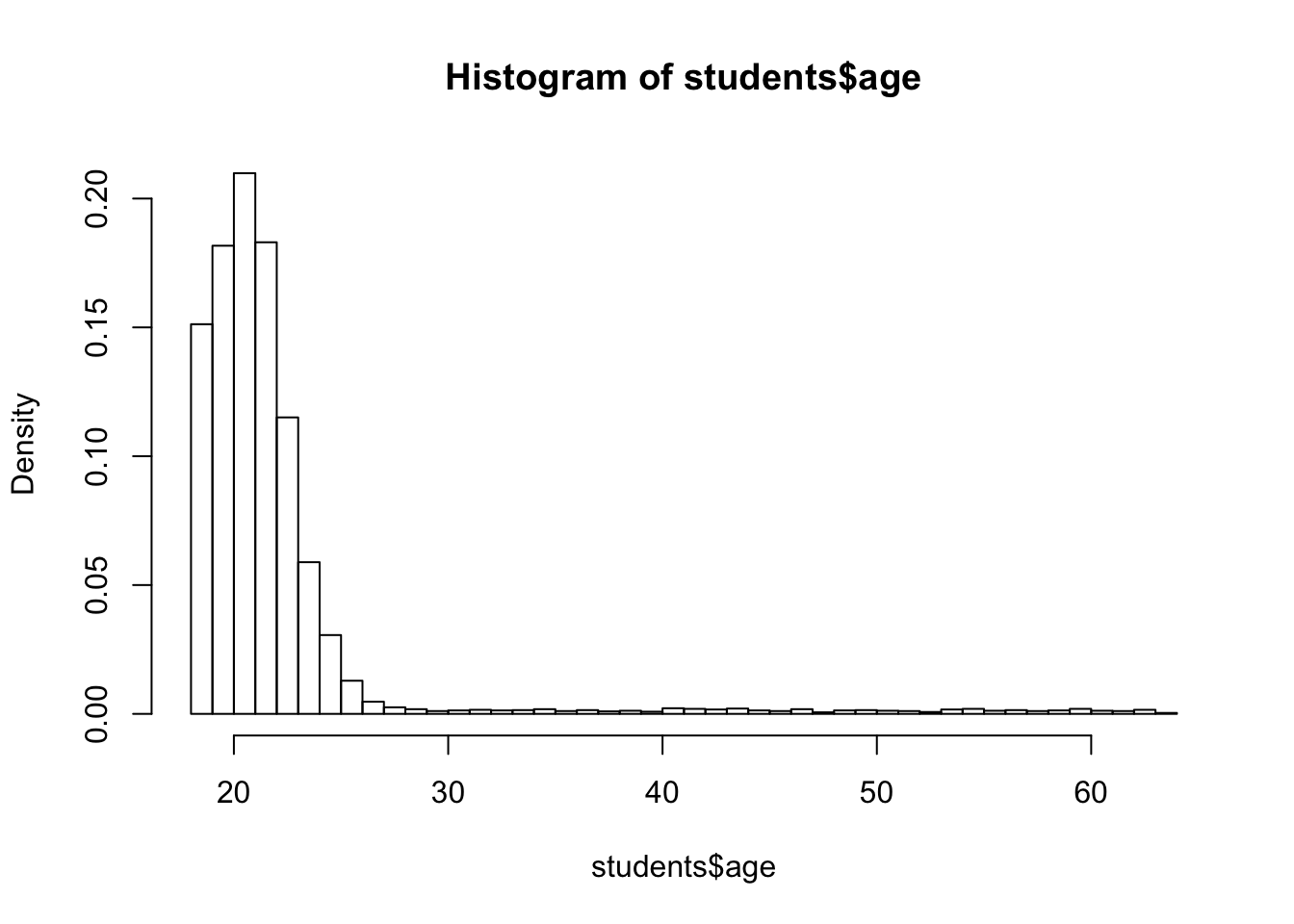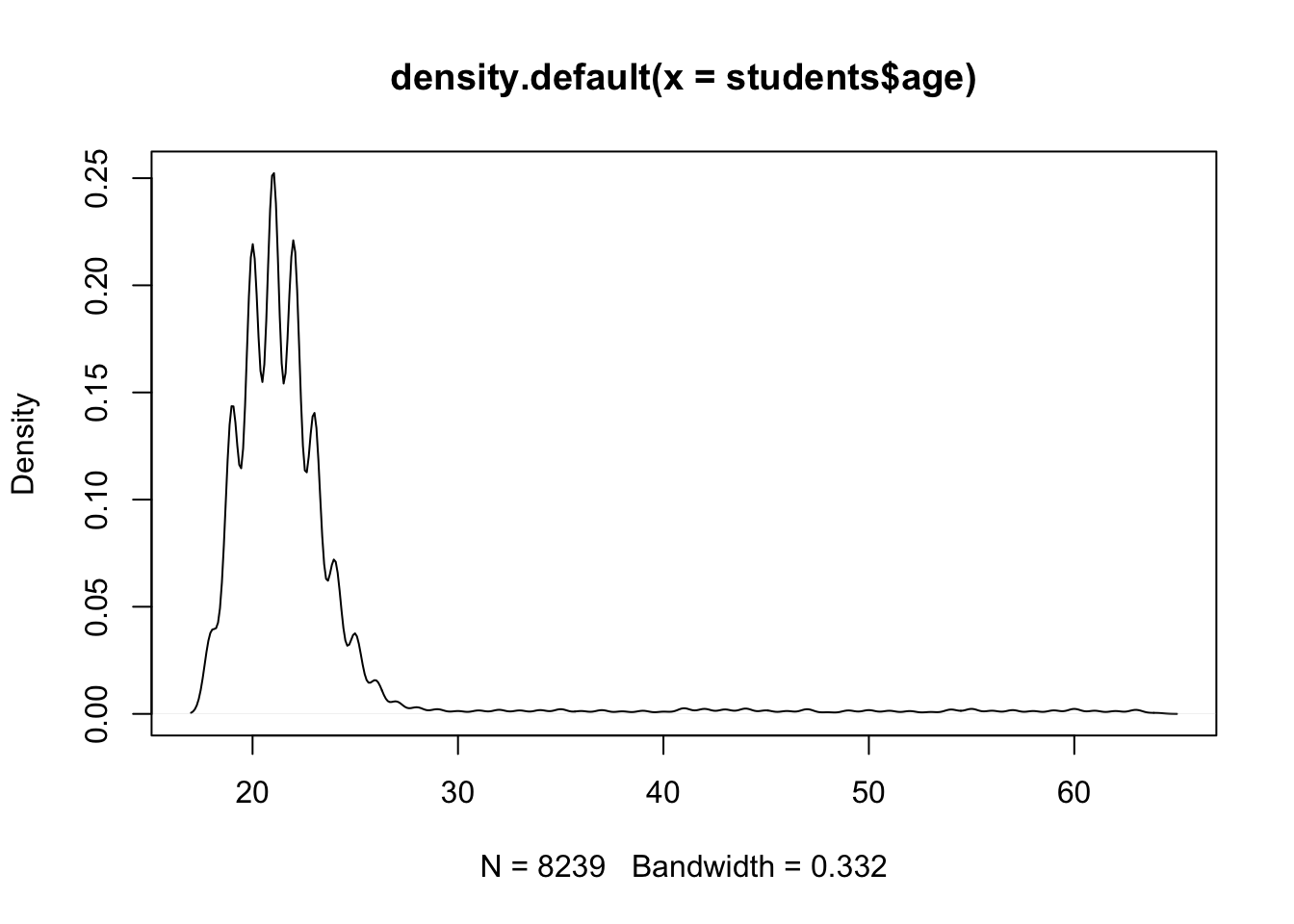In R, graphs are typically created interactively. Creating a new graph by issuing a plotting command, such as `plot()`, `hist()`, `boxplot()`, among others, will typically overwrite a previous graph. In addition one can specify fonts, colors, line styles, axes, reference lines, etc. by specifying graphical parameters. We will walk you through the most important concepts and commands during the subsequent sections.

During this section we will explore a data set called students. You may download the `students.csv` file here.

``````students <- read.csv("https://userpage.fu-berlin.de/soga/200/2010_data_sets/students.csv")
str(students)``````
``````## 'data.frame':    8239 obs. of  16 variables:
##  \$ stud.id        : int  833917 898539 379678 807564 383291 256074 754591 146494 723584 314281 ...
##  \$ name           : Factor w/ 8174 levels "Aarvold, Cindi",..: 2480 4196 7858 5109 5770 5592 1258 162 7221 5240 ...
##  \$ gender         : Factor w/ 2 levels "Female","Male": 1 1 1 2 1 2 1 1 2 1 ...
##  \$ age            : int  19 19 22 19 21 19 21 21 18 18 ...
##  \$ height         : int  160 172 168 183 175 189 156 167 195 165 ...
##  \$ weight         : num  64.8 73 70.6 79.7 71.4 85.8 65.9 65.7 94.4 66 ...
##  \$ religion       : Factor w/ 5 levels "Catholic","Muslim",..: 2 4 5 4 1 1 5 4 4 3 ...
##  \$ nc.score       : num  1.91 1.56 1.24 1.37 1.46 1.34 1.11 2.03 1.29 1.19 ...
##  \$ semester       : Factor w/ 7 levels ">6th","1st","2nd",..: 2 3 4 3 2 3 3 4 4 3 ...
##  \$ major          : Factor w/ 6 levels "Biology","Economics and Finance",..: 5 6 6 3 3 5 5 5 2 3 ...
##  \$ minor          : Factor w/ 6 levels "Biology","Economics and Finance",..: 6 4 4 4 4 4 6 2 3 4 ...
##  \$ score1         : int  NA NA 45 NA NA NA NA 58 57 NA ...
##  \$ score2         : int  NA NA 46 NA NA NA NA 62 67 NA ...
##  \$ online.tutorial: int  0 0 0 0 0 0 0 0 0 0 ...
##  \$ graduated      : int  0 0 0 0 0 0 0 0 0 0 ...
##  \$ salary         : num  NA NA NA NA NA NA NA NA NA NA ...``````

The students data set consists of 8239 rows, each of them representing a particular student, and 16 columns, each of them corresponding to a variable/feature related to that particular student. These self-explaining variables are: stud.id, name, gender, age, height, weight, religion, nc.score, semester, major, minor, score1, score2, online.tutorial, graduated, salary.

#### Histogram

Histograms are created with the `hist()` function. By setting the argument `freq = FALSE` the plot returns probability densities instead of frequencies. The option `breaks` controls the number of bins.

``hist(students\$age)````hist(students\$age, freq = FALSE)````hist(students\$age, freq = FALSE, breaks = 50)``The shape of histograms is strongly affected by the number of bins used, hence it is sometimes useful to plot a kernel density plot instead. In R we can create a kernel density plot by using the `density()` function and by plotting the resulting object.

``plot(density(students\$age))``Note that a density plot is a smoothed histogram. There are many optional parameters in the `density()` function (type `help(density)` into your console for more details). We can easily change the bandwidth parameter by tweaking the `bw` argument.

``plot(density(students\$age, bw = 0.5))``